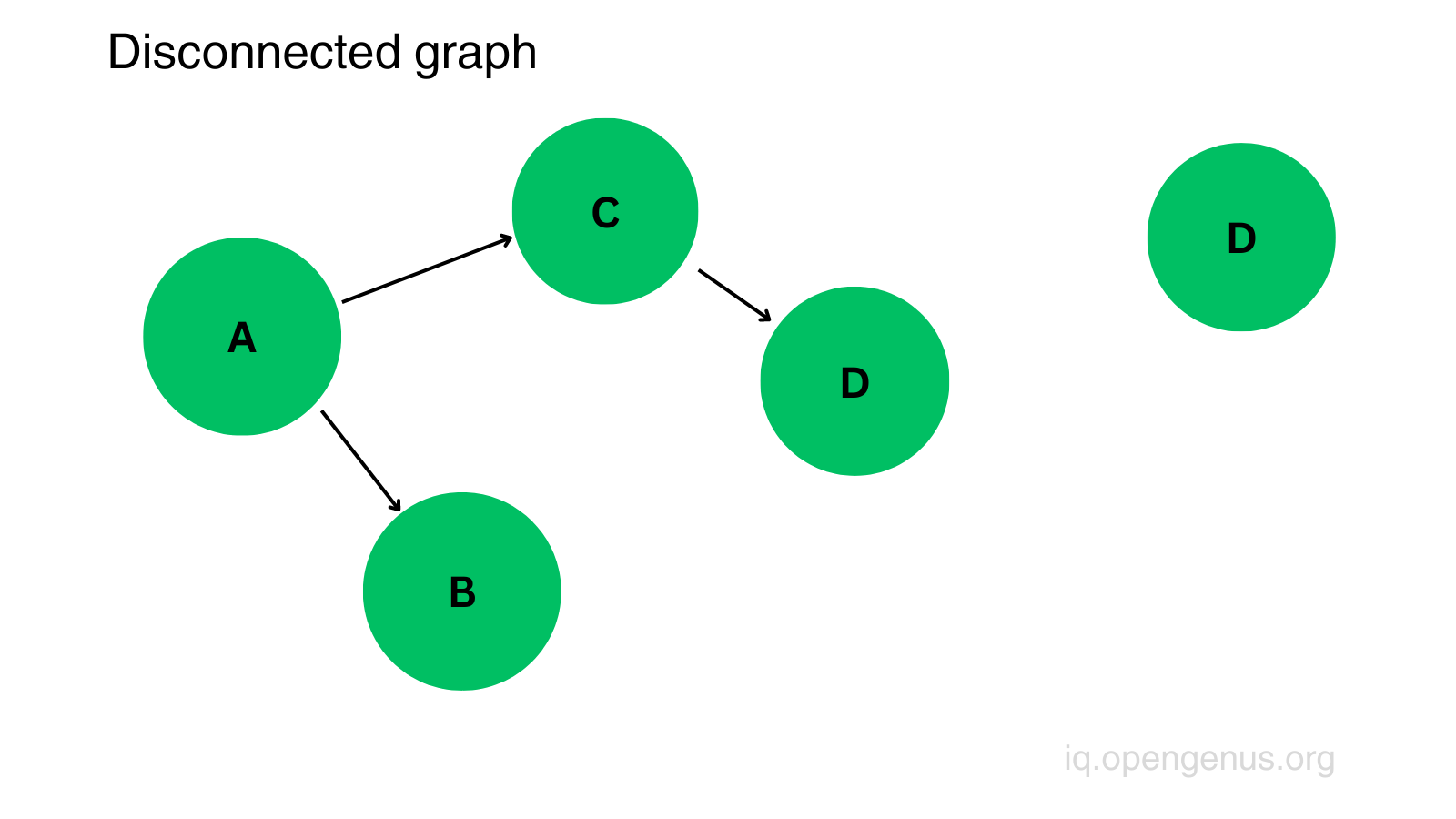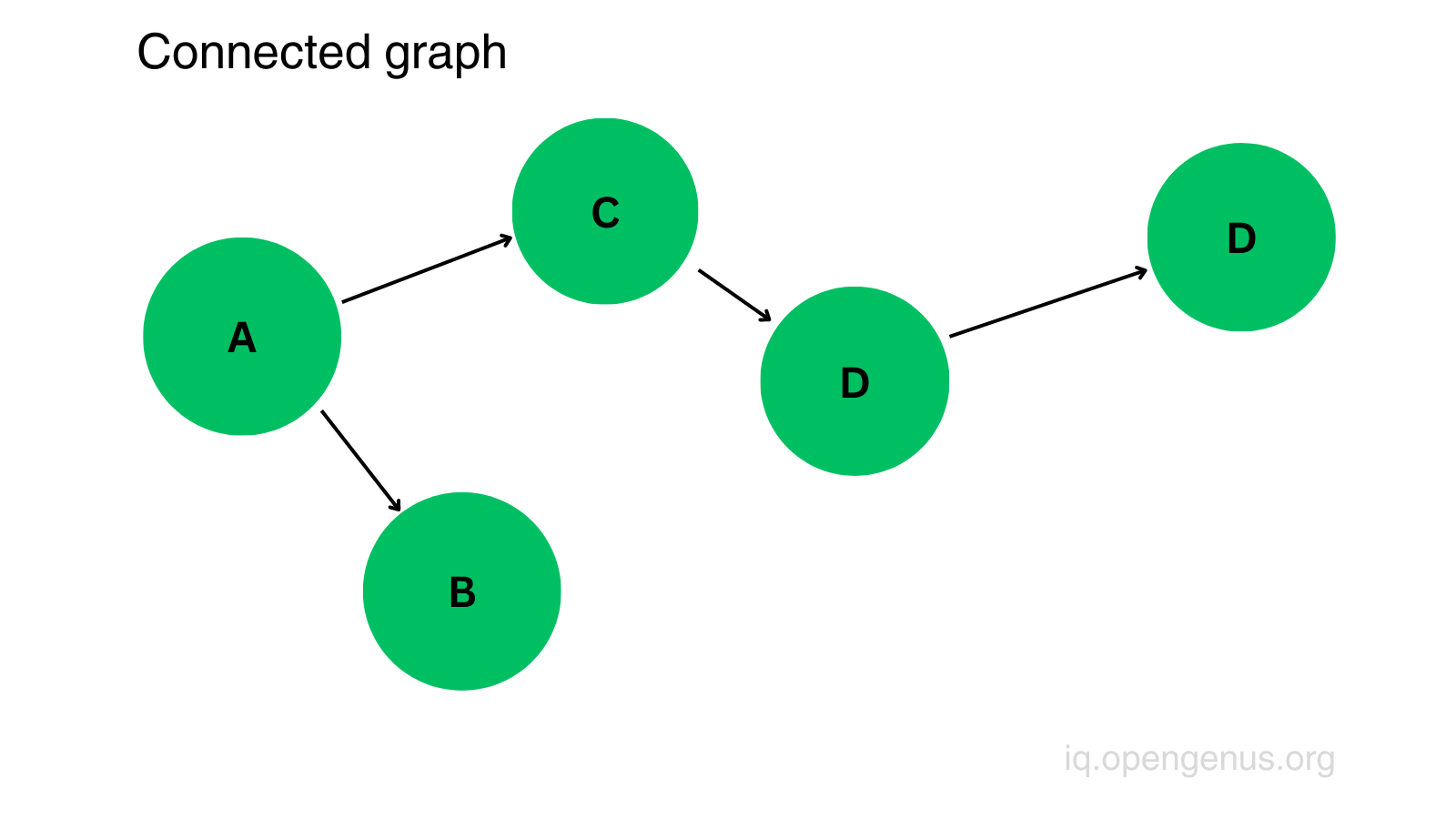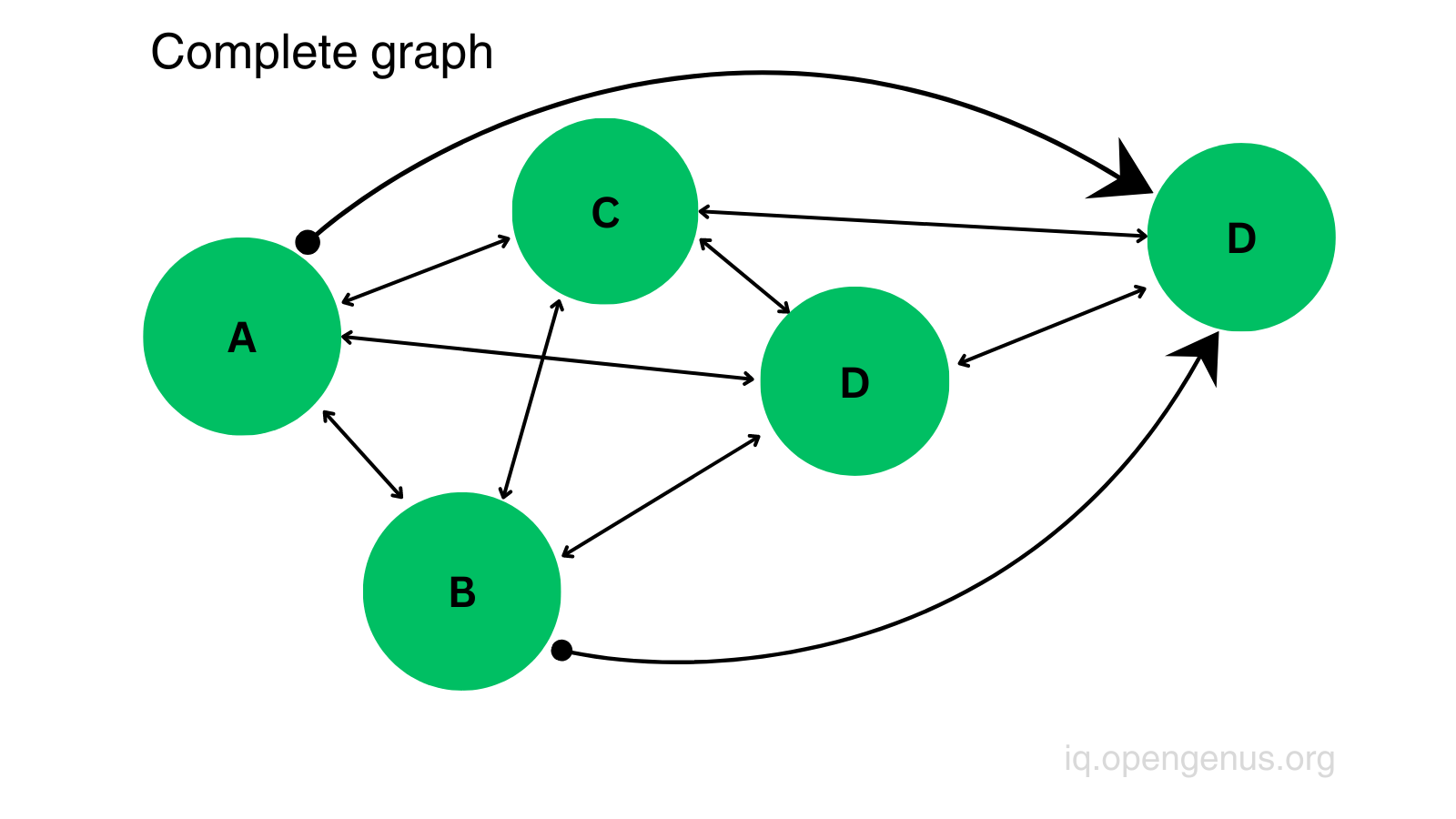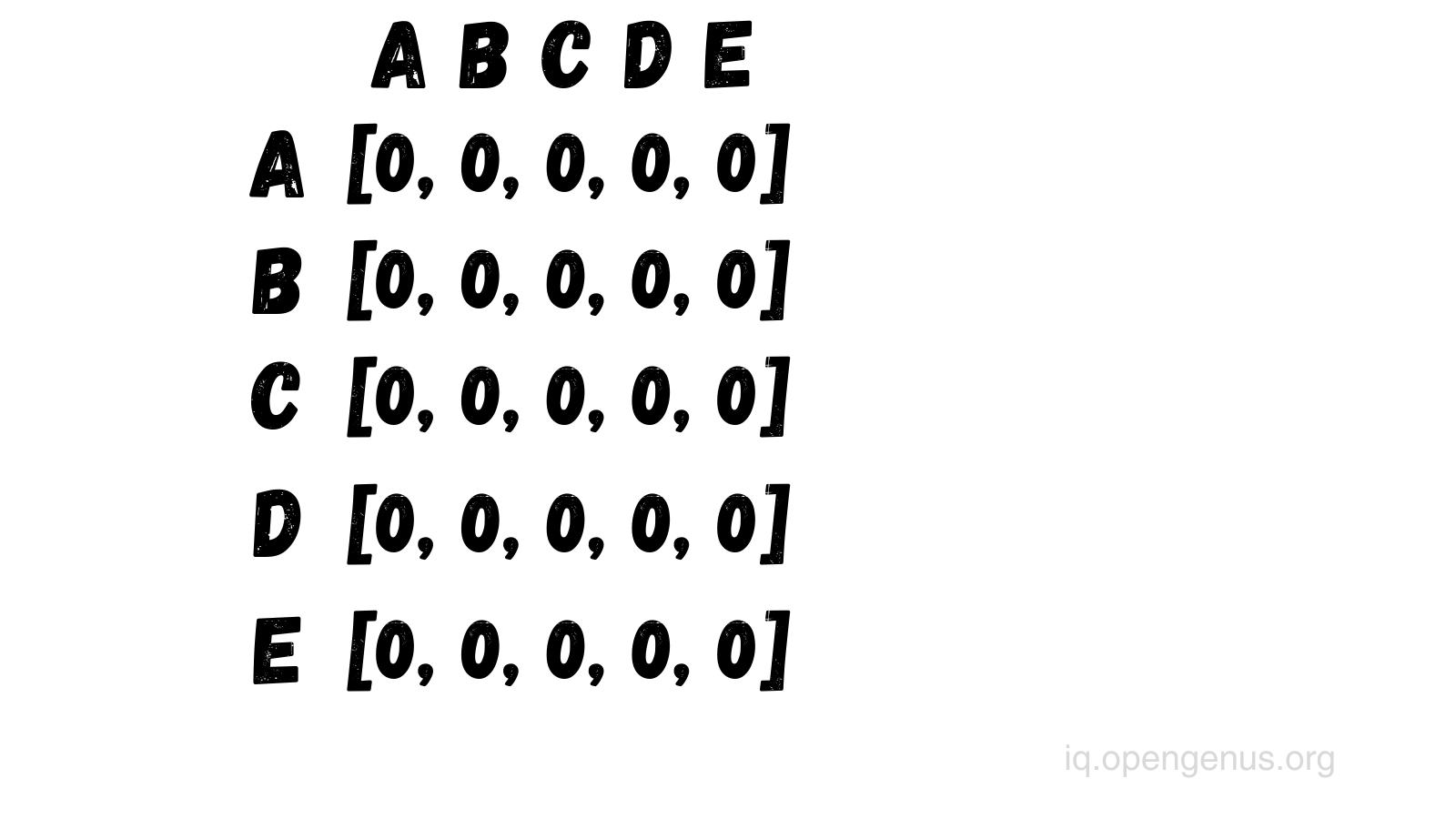# Adjacency list and matrix in JavaScript1. Graphs
4. Conclusion

# 1. Graphs

Graphs are non-linear data structures where a node can have zero or more adjacent (neighbor) elements.
A node is also called vertex (or vertices for plural) and the connection between the nodes are called edges.
Graphs can be:
disconnected: When not all nodes are connected.connected: When all nodes have at least one connection.complete: When all nodes are connected to all other nodes.This list is a data structure to represent connections between nodes in a graph, it is one of the ways to represent a graph.
With this, we can store graph data where each node in the graph is associated with a list (array) of its adjacent (neighboring) nodes. It is implemented using an object or a map.

``````nodeA => [nodeB, nodeC, NodeF, nodeZ, etc...]
``````

In the example above, we can see nodeA and its associated connections.
Let's look at how we can implement an adjacency list using a class.

• Creating a new list (map)
``````class Graph {
constructor() {
this.list = new Map()
}
}

const graph = new Graph() // {}
``````

Everytime we create a new graph, the constructor will create a map ({}). This map will be our adjacency list. Now, let's add different methods in this class.

`````` addNode(node) {
if (!this.list.has(node)) {
this.list.set(node, [])
}
}

graph.addNode(3) // {5 => [], 3 => []}
graph.addNode(9) // {5 => [], 3 => [], 9 => []}
``````

If this node doesn't exists yet, then we add this new node inside the list with an empty array value (where the associated nodes will be stored).

``````  addConnection(node1, node2) {
/* If both nodes exists (for example, in a disconnected graph where not all nodes are connected, where there might be nodes on their own), then we can add a connection from node1 to node2 for example */
if (this.list.has(node1) && this.list.has(node2)) {
this.list.get(node1).push(node2)
} else {
// If node1 exists, then we add node2 inside the node1 array
if (this.list.has(node1) && !this.list.has(node2)) {
// Get the array of node1 and add node2 into it
this.list.get(node1).push(node2)
} else {
// If node1 doesn't exists,
// then we add it to the list and will point to an array containing node2
this.list.set(node1, [node2])
return
}

// If node2 exists, then we add node1 inside the node2 array
if (this.list.has(node2) && !this.list.has(node1)) {
// Get the array of node2 and add node1 into it
this.list.get(node2).push(node1)
} else {
// If node2 doesn't exists,
// then we add it to the list and will point to an array containing node1
this.list.set(node2, [node1])
return
}
}
}
``````

I left comments in the above example to easily understand what each line or block it does. And here is the same example without the comments:

`````` addConnection(node1, node2) {
if (this.list.has(node1) && this.list.has(node2)) {
this.list.get(node1).push(node2)
} else {
if (this.list.has(node1) && !this.list.has(node2)) {
this.list.get(node1).push(node2)
} else {
this.list.set(node1, [node2])
return
}

if (this.list.has(node2) && !this.list.has(node1)) {
this.list.get(node2).push(node1)
} else {
this.list.set(node2, [node1])
return
}
}
}

graph.addConnection(5, 3) // {5 => , 3 => [], 9 => []}
graph.addConnection(1, 9) // {5 => , 3 => [], 9 => [], 1 => }
graph.addConnection(5, 9) // {5 => [3, 9], 3 => [], 9 => [], 1 => }
``````
• Deleting nodes
`````` deleteNode(node) {
if (this.list.has(node)) {
this.list.delete(node)

for (let [n, connections] of this.list) {
const index = connections.indexOf(node)

if (connections.includes(node)) {
connections.splice(index, 1)
}
}
}
}

// Before: {5 => [3, 9], 3 => [], 9 => [], 1 => }
graph.deleteNode(9)
// After: {5 => , 3 => [], 1 => []}
``````

If the node exists in the graph which we want to delete, then we simply call the delete method on the list (which deletes the node). Then we can loop through each node in the list and if the node is found in the array of the associated nodes, then with the splice method we can delete the node as well from the array. You can see, the node number 5 is no longer associated to node number 9, and node n umber 9 is deleted as well.

• Deleting connections
`````` deleteConnection(node1, node2) {
if (this.list.has(node1) && this.list.has(node2)) {
const node1Array = this.list.get(node1)
const node2Array = this.list.get(node2)

const indexOfNode1 = node2Array.indexOf(node1)
const indexOfNode2 = node1Array.indexOf(node2)

if (node1Array.includes(node2)) {
node1Array.splice(indexOfNode2, 1)
}

if (node2Array.includes(node1)) {
node2Array.splice(indexOfNode1, 1)
}
}
}

graph.addConnection(1, 5) // { 5 => [ 3, 1 ], 3 => [], 1 => [ 5 ] }
graph.deleteConnection(5, 1) // { 5 => [ 3 ], 3 => [], 1 => [] }
``````

To delete the connection between two nodes, first we check if the two nodes exist in the graph (if not then there's nothing to delete). Then we get each of the node's associated nodes (the array) and check if node1 is in the node2 array and vice versa. If they are inside the array, then they will get deleted with the splice method.

• Checking if two nodes are connected
`````` checkConnection(node1, node2) {
if (this.list.has(node1) && this.list.has(node2)) {
const node1Array = this.list.get(node1)
const node2Array = this.list.get(node2)

if (node1Array.includes(node2) && node2Array.includes(node1)) {
console.log('true')
return
}
console.log('false')
}
}

graph.addConnection(3, 5) // { 5 => [ 3 ], 3 => , 1 => [] }
graph.checkConnection(5, 3) // true
graph.checkConnection(5, 1) // false
``````

This example shows if two nodes are connected with each other, checks if the connections are bi-directional (node1 <=> node2). These graphs are called undirected. If they are connected, then we log true to the console and return, otherwise it will log false to the console. Note that if we omit the return keyword, then false would be logged to the console as well no matter if the nodes are connected or not.
We could have another statement to check if two nodes are connected but with one direction (node1 => node2). These graphs are called directed or di-graph. So either node1 is connected to node2, or node2 is connected to node1.
Then the code would look something like this:

`````` checkConnection(node1, node2) {
if (this.list.has(node1) && this.list.has(node2)) {
const node1Array = this.list.get(node1)
const node2Array = this.list.get(node2)

if (node1Array.includes(node2)) {
console.log('true')
return
}

if (node2Array.includes(node1)) {
console.log('true')
return
}
console.log('false')
}
}
``````
• Printing list
`````` printList() {
for (let [node, connections] of this.list) {
console.log(node + "->" + connections)
}
}

graph.printList() // 5 -> 3
3 -> 5
1 ->
``````

To print the list, we need to loop through each node and just print out (log) their associated nodes.

Another way of representing a graph is using a two-dimensional array. Where the nodes are connected, we add 1, if they are not then we add 0 or -.• Creating a new matrix
``````class Graph {
constructor(numOfNodes) {
this.numOfNodes = numOfNodes;
this.matrix = []

for (let i = 0; i < numOfNodes; i++) {
this.matrix[i] = [];
for (let j = 0; j < numOfNodes; j++) {
this.matrix[i][j] = 0;
}
}
}

const graph = new Graph(5)
/*
numOfNodes: 5,
matrix: [
[ 0, 0, 0, 0, 0 ],
[ 0, 0, 0, 0, 0 ],
[ 0, 0, 0, 0, 0 ],
[ 0, 0, 0, 0, 0 ],
[ 0, 0, 0, 0, 0 ]
]
*/
``````

To create a new matrix we need to pass in a number (number of nodes).
Then, with a for loop we create the two-dimensional array. In this example, we passed in the number 5, so we created 5 arrays with 5 elements.

`````` addConnections(node1, node2) {
if (node1 >= 0 && node1 < this.numOfNodes && node2 >= 0 && node2 < this.numOfNodes) {
this.matrix[node1][node2] = 1
}
}

/*
[ 0, 0, 1, 0, 0 ],
[ 0, 0, 0, 1, 0 ],
[ 0, 0, 0, 0, 0 ],
[ 0, 1, 0, 0, 0 ],
[ 0, 0, 0, 0, 0 ]
*/
``````

Since we already created the nodes (the arrays), we only need to add connections. We check if the two nodes we want to connect are greater or equal than 0 and if they are smaller than the number of nodes. If yes, then we add number 1 in their intersection.

• Deleting connections
``````  deleteConnections(node1, node2) {
if (node1 >= 0 && node1 < this.numOfNodes && node2 >= 0 && node2 < this.numOfNodes) {
this.matrix[node1][node2] = 0
}
}

graph.deleteConnections(0, 2)
/*
[ 0, 0, 0, 0, 0 ],
[ 0, 0, 0, 1, 0 ],
[ 0, 0, 0, 0, 0 ],
[ 0, 1, 0, 0, 0 ],
[ 0, 0, 0, 0, 0 ]
*/
``````

This method is very similar to the addingConnections method, the only thing we need to change here is to replace number 1 with the number 0.

• Deleting nodes
``````deleteNode(node) {
if (node >= 0 && node < this.numOfNodes) {
this.matrix.splice(node, 1);
this.numOfNodes--;

for (let i = 0; i < this.numOfNodes; i++) {
this.matrix[i].splice(node, 1)
}
}
}

graph.deleteNode(2)
/*
numOfNodes: 4,
matrix: [
[ 0, 0, 0, 0 ],
[ 0, 0, 1, 0 ],
[ 0, 1, 0, 0 ],
[ 0, 0, 0, 0 ]
]
*/
``````

If we want to delete nodes, then we can do that with the splice method and we also need to decrement the number of nodes by one. Also, we need to delete one element from each array.

• Checking if two nodes are connected
`````` checkConnection(node1, node2) {

if (this.matrix[node1][node2] === 1) {
console.log('true')
return
}
console.log('false')
}

graph.checkConnection(2, 1) // true
graph.checkConnection(2, 0) // false
``````

This is one example of checking if two nodes are connected.
Of course, we could check if they are connected bi-directionally:

`````` checkConnection(node1, node2) {

if (this.matrix[node1][node2] === 1) {
console.log('true')
return
}

if (this.matrix[node2][node1] === 1) {
console.log('true')
return
}

console.log('false')
}
``````
• Printing the matrix
``````printMatrix() {
for (let i = 0; i < this.numOfNodes; i++) {
const row = this.matrix[i].join(' ')
console.log(row)
}
}

graph.printMatrix()
/*
0 0 0 0 0
0 0 0 1 0
0 1 0 0 0
0 0 0 0 0
*/
``````

Here, we just print out each node (each array) in one separate line.

# 4. Conclusion

Now you know how to implement an adjacency list and matrix to store graph data. You see, my examples were simple and the methods are not complex at all. If you have to work with graphs or graph related data, then it's up to you how you want to represent the graph, using a list or a two-dimensional array.

Here you can check out the full example: